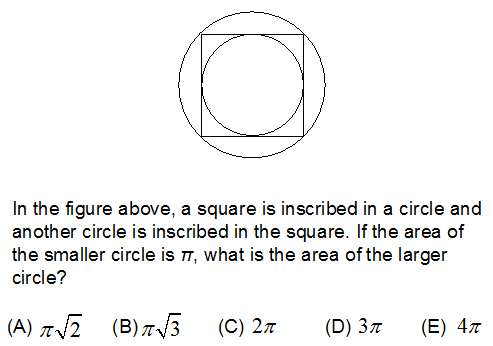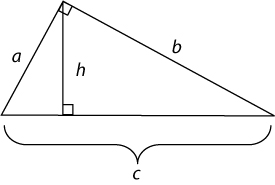## Articles tagged "geometry"

### What’s Tested on GRE MathGRE Math is a bit like high school math, without some of the hardest parts: for instance, you don’t have to write proofs or show your work! Here’s a quick rundown of the GRE Math skills required to conquer the Quant section, along with some of our best GRE Math tips. Read more

### GRE Geometry: The Impossible TaskIn one of my recent classes, I told the students “You’ll never know how to answer a geometry question.”  The reaction was fairly predictable: “Why would you say that?!?  That’s so discouraging!!”

Of course, I certainly was NOT trying to discourage them.  I used that statement to illustrate that geometry questions are often a type of quantitative question that can feel immensely frustrating!  You know what shape you have, you know what quantity the question wants, but you have no idea how to solve for that quantity.

This is what I meant when I said you’ll never know how to answer these questions. That “leap” to the correct answer is impossible.  You can’t get to the answer in one step, but that’s all right: you’re not supposed to!

(An important aside: if you’ve read my post regarding calculation v. principle on the GRE, you should be aware that I am discussing the calculation heavy geometry questions in this post.)

The efficient, effective approach to a calculation-based geometry question is NOT to try and jump to the final answer, but instead to simply move to the next “piece”.  For example, let’s say a geometry question gives me an isosceles triangle with two angles equaling x.  I don’t know what x is, and I don’t know how to use it to find the answer to the question.  But I DO know that the third angle is 180-2x.

That’s the game.  Find the next little piece.  And the piece after that.  And the piece after that.  Let’s see an example.The correct response to this problem is “Bu-whah???  I know nothing about the large circle!”

But you do know the area of the smaller circle.  What piece will that give you?  Ok, you say, area gives me the radius.  A = pi*r^2, so pi = pi*r^2, so r^2 = 1, so r = 1.  Done, and let’s put that in the diagram.Each week, we post a new Challenge Problem for you to attempt. If you submit the correct answer, you will be entered into that week’s drawing for two free Manhattan Prep GRE Strategy Guides.